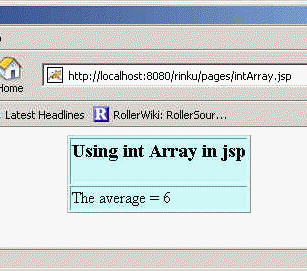Ads

Tutorials

# int Array

Array is a collection of same data type. Suppose if we have declared an array of type int then the array will take only the int values and not any other data types.

Array is a collection of same data type. Suppose if we have declared an array of type int then the array will take only the int values and not any other data types.

# int Array

Array is a collection of same data type. Suppose if we have declared an array of type int then the array will take only the int values and not any other data types. We can find find out the length of  the variable by using the variable length.

The code of the program is given below:

 ``` Using int Array in jsp ``` ```

Using int Array in jsp

<% int array[] = {0, 2, 4, 8, 16}, sum = 0;``` ``` for (int i = 0; i < array.length;i++){ ``` ``` sum=sum+array[i]; } out.println("The average = " + sum / array.length); %>
```

Output of  the Program:Download this example.

Ads

Ads